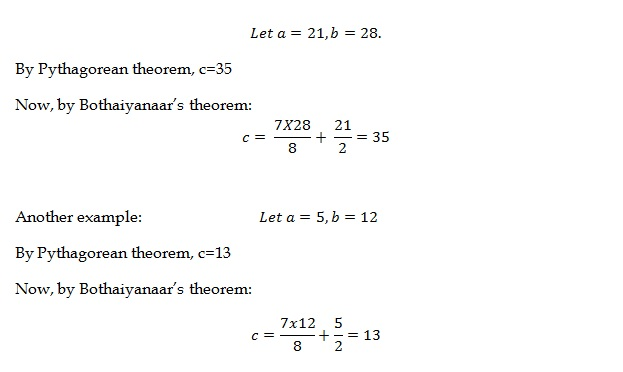# Bothaiyanaar Alternative to Pythagorean Theorem

Bothaiyanaar’s Alternative to Pythagorean Theorem

It was quite accidentally, when reading about Pythagorean theorem, that I stumbled upon this amazingly simpler theorem which stands as an alternative to Pythagorean theorem. This was actually written by a Tamil poet – Bothaiyanaar – and is useful while calculating the hypotenuse of a right angled triangle. Note that this theorem works well when the numbers involved are whole numbers. When the hypotenuse is not a whole number, the theorem seems to be giving not accurate, but approximate values. The following is a stanza from Bothaiyanaar’s poem (in Tamil script[ref]http://ramsundarvijayraj.blogspot.in/2011/12/bothaiyanaar-pythagoras-theorem.html[/ref]):

“ஓடும் நீளம் தனை ஒரேஎட்டுக்
கூறு ஆக்கி கூறிலே ஒன்றைத்
தள்ளி குன்றத்தில் பாதியாய்ச் சேர்த்தால்
வருவது கர்ணம் தானே”

This [ref]www.durofy.com/alternate-pythagoras-theorem[/ref] means: Divide the distance you’ve run (longer side) into 8 equal parts and discard one part from it. Then if you add the half the height to that, you’ll get Hypotenuse.

The advantage of the theorem is that we need not perform the square root function, which might be a hazard for the little school students. That way, this works fine for them. Let’s see how it works:

Consider two smaller sides, say a and b, of a right-angled triangle. Let$a \leq b$. Then,$hyp = \frac{7b}{8}+\frac{a}{2}$

Let’s discuss two examples now:Further, this trick seems to be applicable for the normal pythagorean triplets of the form (3x, 4x, 5x) and (5x, 12x, 13x). It doesn’t seem to be okay for (7x, 24x, 25x), where it gives not an accurate answer.

Thanks to Manish Bansal for a quick proof as to why this works, that’s given below:

Let’s assume that this theorem is true for all whole numbers, as far as a right angled triangle is concerned. On comparing the hypotenuse of this theorem and traditional Pythagorean theorem:$\Rightarrow a^{2}+b^{2} = \left ( \frac{7b}{8} + \frac{a}{2} \right )^{2}$$\Rightarrow a^{2}+b^{2} = \frac{49b^{2}}{64}+\frac{7ab}{8}+\frac{a^{2}}{4}$

Multiplying both sides by 64,$\Rightarrow 64a^{2} + 64b^{2} = 49b^{2}+56ab+16a^{2}$$\Rightarrow 48 a^{2}+15b^{2}=56ab$

Dividing both sides by$ab$,$\Rightarrow 48\frac{a}{b}+15\frac{b}{a}=56$

Let z = a/b.$\Rightarrow 48z+\frac{15}{z}=56$

Multiplying by z,$\Rightarrow 48z^{2}-56z+15=0$

Applying the basic equation to solve the above quadratic equation,$\Rightarrow z=\frac{56\pm \sqrt{56\times 56-4\times 48\times 15}}{2\times 48}$$\Rightarrow z=\frac{5}{12}, \frac{3}{4}$

This shows that the ratio a/b, is either 3/4 or 5/12. Only then, it will give proper results for the hypotenuse. If the ratio is 7/24, this theorem will not give accurate result as that’s not a root of the above quadratic equation. The theorem as such might not look promising, but it is nice to have a thought on the simplicity of this theorem, though having limited application.

Thanks,

Arunanand T A